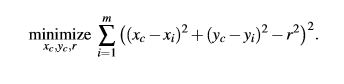# Only scalar quadratic forms can be specified in CVX :minimize ((norm(- b + A*z )))

given x and y data points, I am trying to minimize the function shown in the image, but getting below error.

Disciplined convex programming error:
Only scalar quadratic forms can be specified in CVX ![cvxprob1|344x84]

``````> cvx_begin
> variable x_c(1)
> variable y_c(1)
> variable r(1)
> variable z(3,1)
> variable A(50,3)
> variable b(50,1)
> z=[x_c ; y_c ; r];
> A=[x_c-2*x y_c-2*y -r*ones(50,1)];
> b=-((x.*x)+(y.*y));
> minimize ((norm(- b + A*z )))
> cvx_end
> t = linspace(0, 2*pi, 1000);
> plot(x, y, 'o', r*cos(t) + x_c, r*sin(t) + y_c, '-');
``````

could you please suggest some help.

I can’t see the image, so I don’t know what problem you are trying to solve.

Is the problem (objective function in this case) convex? if it is, you must follow CVX’s rules. `A*z` does not follow CVX’s rule (CVX can not determine ti to be convex or concave), and even if it did, it is nonlinear, and therefore invalid as an argument to `norm`.

Also, `z, A` should not be declared as variables, because they are expressions built up from CVX variables. `b` should not be declared as a variable, because it apparently is just a constant (presuming `x` and `y` are constants).

It seems apparent you need to read or re-read the CVX Users’ Guide.Thank you I will check once again.Above is the problem I am trying to solve.

The `-r^2` looks rather fishy relative to convexity, even if you use a variable `r_squared`. Have you proven convexity? I will mark this as non-convex unless you show otherwise.

Are all your difficulties now resolved?